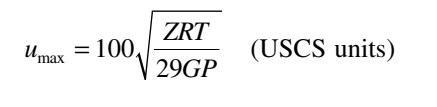# Erosional Velocity of a Gas Pipeline

### The gas velocity is directly related to the flow rate. As flow rate increases, so does the gas velocity. As the velocity increases, vibration and noise are evident. In addition, higher velocities will cause erosion of the pipe interior over a long period of time.

The upper limit of the gas velocity is usually calculated approximately from the following equation:Erosional Velocity Formula

Where,

umax = maximum or erosional velocity, ft/s
ρ = gas density at flowing temperature, lb/ft
3

Since the gas density ρ may be expressed in terms of pressure and temperature, the maximum velocity can be rewritten as,Where,

Z = compressibility factor of gas, dimensionless
R = gas constant = 10.73 ft3 psia/lb-moleR
T = gas temperature, °R
G = gas gravity (air = 1.00)
P = gas pressure, psia

Usually, an acceptable operational velocity is 50% of the above.